# Class 7 Maths NCERT Solutions for Chapter – 5 Lines and Angles EX – 5.1

## Lines and Angles

Question 1.
Find the complement of each of the following angles :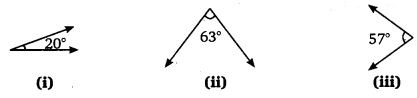Solution:
Since, the sum of the measures of an angle and its complement is 90°, therefore,

1. The complement of an angle of measure 20° is the angle of (90°-20°), f.e., 70°.
2. The complement of an angle of measure 63° is the angle of (90°-63°), i.e., 27°.
3. The complement of an angle of measure 57° is the angle of (90°-57°), i.e., 33°.

Question 2.
Find the supplement of each of the following angles :Solution:
Since, the sum of the measures of an angle and its supplement is 180°, therefore,

1. The supplement of an angle of measvjre 105° is the angle of (180°-105°), i.e., 75°.
2. The supplement of an angle of measure 87° is the angle of (180°-87°), i.e., 93°.
3. The supplement of an angle of measure 154° is the angle of (180°-154°), i.e., 26°.

Question 3.
Identify which of the following pairs of angles are complementary and which are supplementary :

1. 65°, 115°
2. 63°, 27°
3. 112°, 68°
4. 130°, 50°
5. 45°, 45°
6. 80°, 10°

Solution:

1. Since, 65°+ 115° = 180°
So, this pair of angles is supplementary.
2. Since, 63°+ 27° = 90°
So, this pair of angles is complementary.
3. Since, 112° + 68° = 1800
So, this pair of angles is supplementary.
4. Since, 130°+50° = 180°
So, this pair of angles is supplementary.
5. Since, 45°+ 45° = 90°
So, this pair of angles is complementary.
6. Since, 80°+ 10° = 90°
So, this pair of angles is complementary.

Question 4.
Find the angle which is equal to its complement.

Solution:
Let the measure of the angle be x°. Then, the measure of its complement is given to be x°.
Since, the sum of the measures of an angle and its complement is 90°, therefore,
x° + x° = 90°
⇒ 2x° = 90°
⇒ x° = 45°
Thus, the required angle is 45°.

Question 5.
Find the angle which is equal to its supplement.

Solution:
Let the measure of the angle be x°. Then,
measure of its supplement = x°
Since, the sum of the measures of an angle and its supplement is 180°, therefore,
x° + x° = 180°
⇒ 2x° =180°
⇒ x° = 90°
Hence, the required angle is 90°.

Question 6.
In the given figure, ∠1 and ∠2 are supplementary angles. If ∠1 is decreased, what changes should take place in ∠2 so that both the angles still remain supplementary?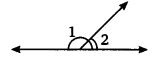Solution:
∠2 will increase with the same measure as the decrease in ∠1.

Question 7.
Can two angles be supplementary if both of them are :

1. acute?
2. obtuse?
3. right?

Solution:

1. No
2. No
3. Yes

Question 8.
An angle is greater than 45°. Is its complementary angle greater than 45° or equal to 45° or less than 45°?

Solution:
Since, the sum of the measure of ah angle and its complement is 90°.
∴ The complement of an angle of measures 45° + x°, where x > 0 is the angle of [90° – (45° + x°)] = 90° – 45° – x°= 45° – x°.
Clearly, 45° + x° > 45° – x°
Hence, the complement of an angle > 45° is less than 45°.

Question 9.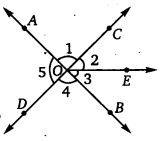1. Is ∠1 adjacent to ∠2 ?
2. Is ∠AOC adjacent to ∠AOE?
3. Do ∠COE and ∠EOD form a linear pair?
4. Are ∠BOD and ∠DOA supplementary?
5. Is ∠1 vertically opposite to Z4?
6. What is the vertically opposite angle of ∠5

Solution:

1. Yes
2. No
3. Yes
4. Yes
5. Yes
6. ∠2 + ∠3 = ∠COB

Question 10.
Indicate which pairs of angles are :

1. Vertically opposite angles.
2. Linear pairs.

Solution:

1. Pair of vertically opposite angles are ∠1, ∠4; ∠5, ∠2 + ∠3.
2. Pair of linear angles are ∠1, ∠5; ∠4, ∠5.

Question 11.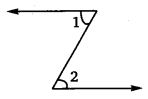Solution:
∠1 is not adjacent to ∠2 because they have no common vertex.

Question 12.
Find the values of the angles x, y and z in each of the following?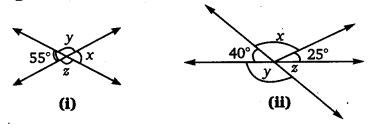Solution:

Question 13.
Fill in the blanks :
(i) If two angles are complementary, then the sum of their measures is __________
(ii) If two angles are supplementary, then the sum of their measures is __________
(iii) Two angles forming a linear pair are __________
(iv) If two adjacent angles are supplementary, they form a __________
(v) If two lines intersect at a point, then the vertically opposite angles are always __________
(vi) If two lines intersect at a point, and if one pair of vertically opposite angles are acute angles, then the other pair of vertically opposite angles are __________

Solution:
(i) 90°
(ii) 180°
(iii) supplementary
(iv) linear pair
(v) equal
(vi) obtuse angles

Question 14.
In the adjoining figure, name the following pairs of angles :1. Obtuse vertically opposite angles.
3. Equal supplementary angles.
4. Unequal supplementary angles.
5. Adjacent angles that do not form a linear pair.

Solution:

1. Obtuse vertically opposite angles are ∠AOD and ∠BOC.
2. Adjacent complementary angles are ∠BOA and ∠AOE.
3. Equal supplementary angles are ∠BOE and ∠EOD.
4. Unequal supplementary angles are ∠BOA and ∠AOD, ∠BOC and ∠COD, ∠EOA and ∠EOC.
5. Adjacent angles that do not form a linear pair are ∠AOB and ∠AOE, ∠AOE and ∠EOD; ∠EOD and ∠COD.